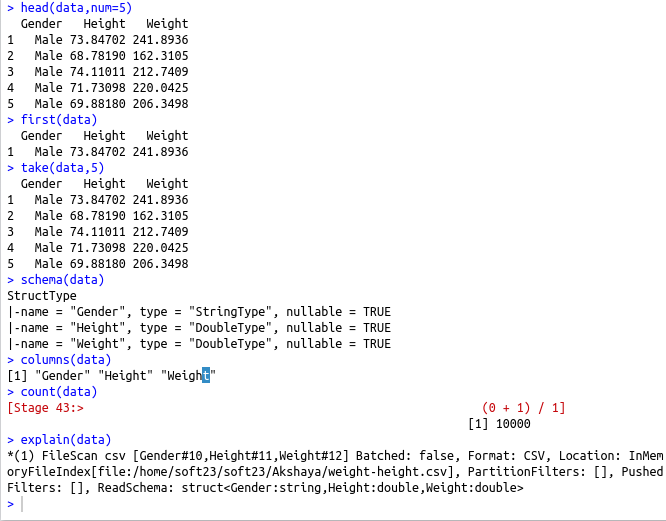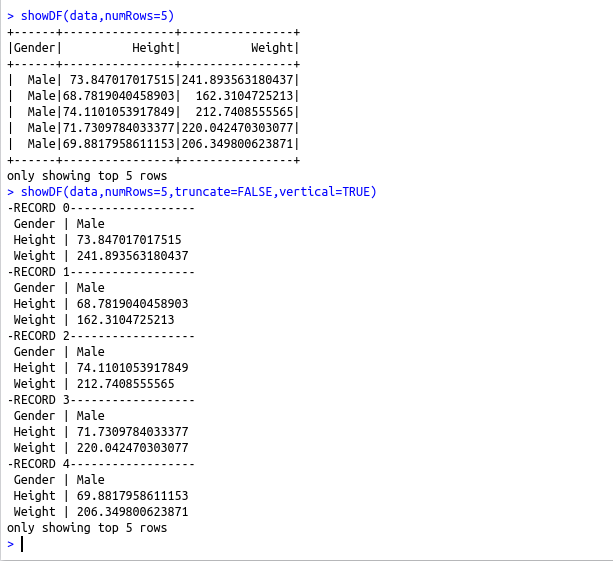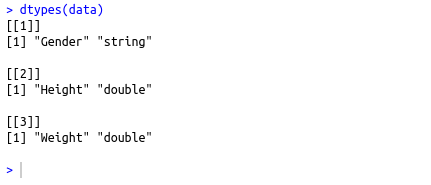• #5, First Floor, 4th Street Dr. Subbarayan Nagar Kodambakkam, Chennai-600 024 Landmark : Samiyar Madam
• pro@slogix.in
• +91- 81240 01111

### How to inspect a Spark Data Frame using R?

###### Description

To inspect Spark Data Frame using R

#### Functions used :

dtypes(data) – To inspect the Spark data frame
showDF(data,numRows,truncate,vertical) – To print the first numRows of a spark dataframe(numRows=no of rows to show,truncate=False to not to truncate the dataframe,vertical=TRUE to print the dataframe vertically)
head(data,num=10) – Returns the first num rows of a SparkDataFrame as a R data.frame
first(data) – Returns the first row of a SparkDataFrame
take(data,5) -m Take the first NUM rows of a SparkDataFrame and return the results as a R data.frame
schema(data) – To get the schema of the data
columns(data) – To get the columns of a spark dataframe
count(data) – To count the number of rows in spark data frame
explain(data) – To print the logical and physical Catalyst plans to the console for debugging

###### Process
• Set up spark home
• Load the spark library
• Initialize the spark context
• Load the data set
• Inspect the data frame using the pre-defined functions
###### Sapmle Code

#Set up spark home
.libPaths(c(file.path(Sys.getenv(“SPARK_HOME”), “R”, “lib”), .libPaths()))
library(SparkR)
#Initialize the Spark Context
#To run spark in a local node give master=”local”
sc #Start the SparkSQL Context
sqlContext #Load the data set
data = read.df(“file:///…/weight-height.csv”,”csv”,header = “true”, inferSchema = “true”, na.strings = “NA”)
#To inspect the Spark data frame
dtypes(data)
#To print the first numRows of a spark dataframe
showDF(data,numRows=5)
showDF(data,numRows=5,truncate=FALSE,vertical=TRUE)
#Return the first num rows of a SparkDataFrame as a R data.frame
#Return the first row of a SparkDataFrame
first(data)
#Take the first NUM rows of a SparkDataFrame and return the results as a R data.frame
take(data,5)
#To get the schema of the data
schema(data)
#To get the columns of a spark dataframe
columns(data)
#To count the number of rows in spark data frame
count(data)
#To print the logical and physical Catalyst plans to the console for debugging
explain(data)

###### Screenshots